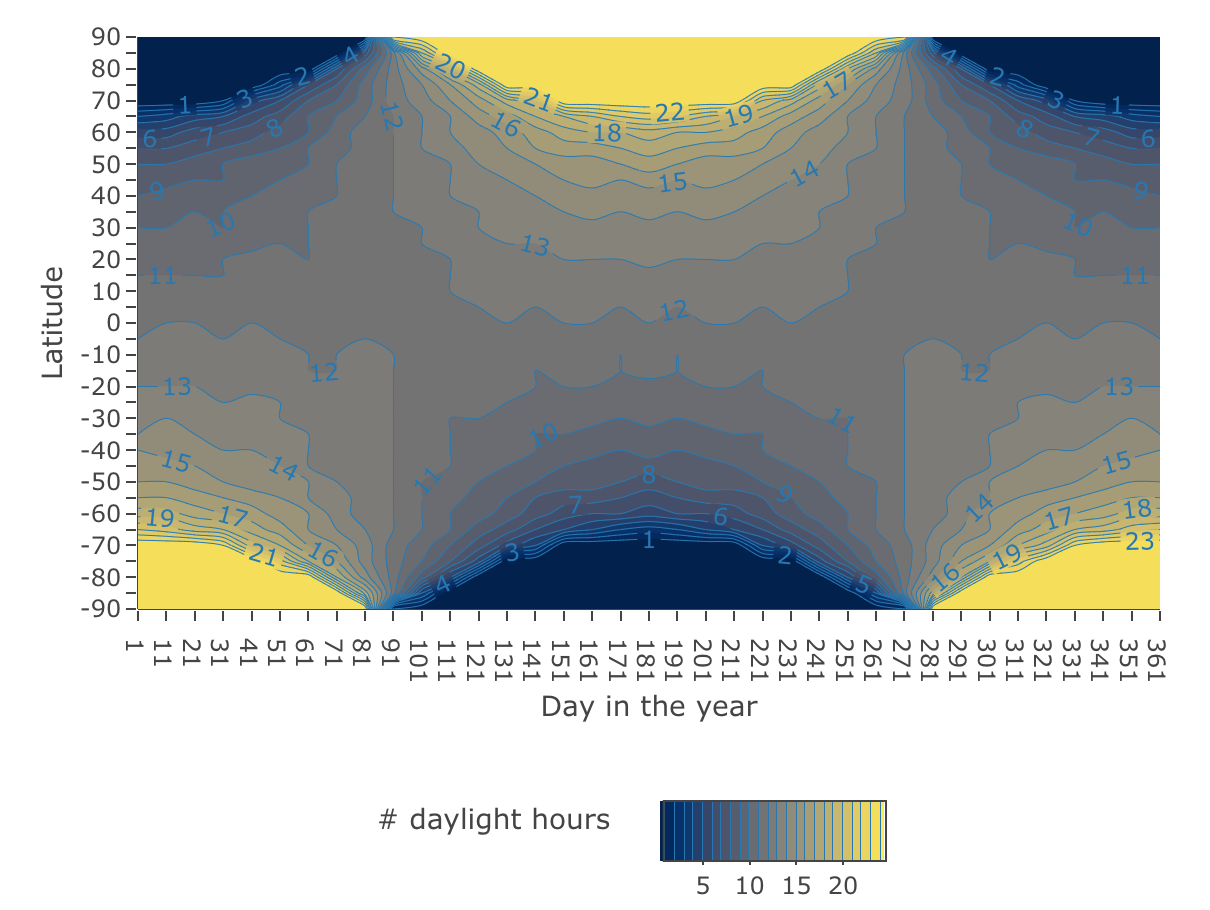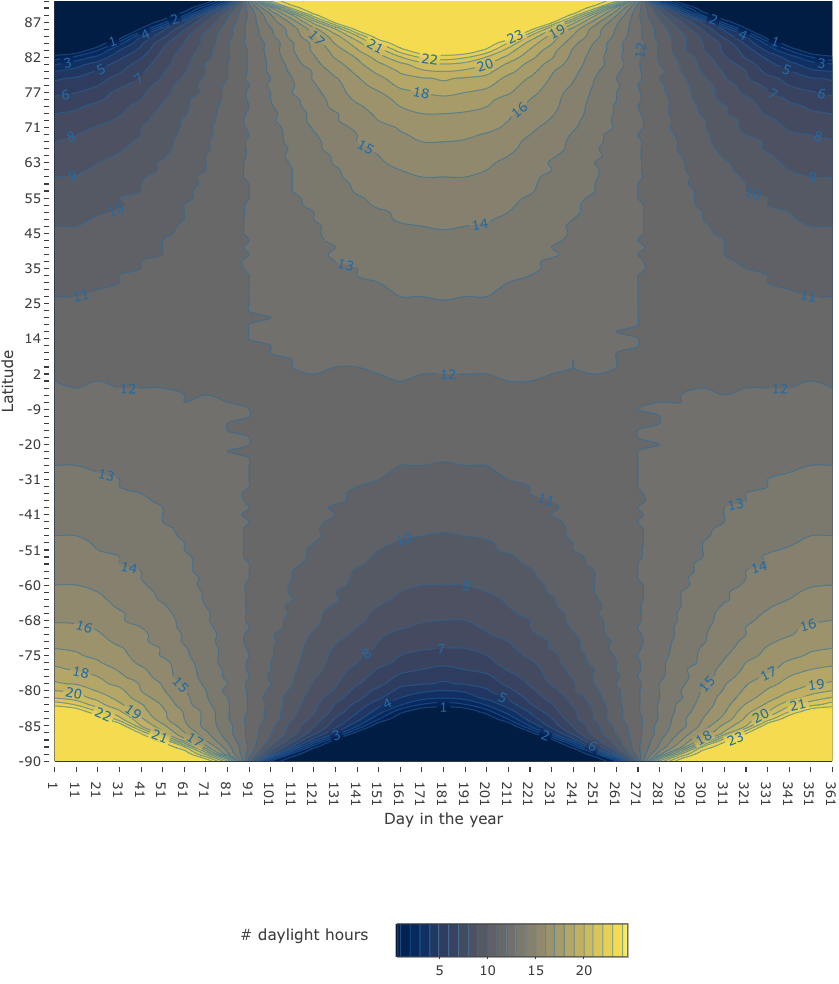# Daytime length variations with latitude and season (3/3)

It is time to conclude this series, but not before making some closing arguments.

# Resolution

It is possible to increase the resolution of the plot by decreasing the length of the step for the different dimensions: latitude, longitude and days in the year. For instance, the values (lat_step = 5, lon_step = 1, year_step = 10) result in the following plot 1.

• Points in the sphere (N) = num_lat_pts $$\times$$ num_lon_pts = 1776
• Days in the year = 37Figure 1: Figure 1: Daylength by latitude and day in the year for ( lat_step = 5, lon_step = 1, year_step = 10)

## Is there a better way of generating the grid?

While it is possible to smooth the contours by increasing the number of points in the grid at the expense of computation and rendering time, something struck me: the resolution around the poles is higher than at the Equator.

The number of points in each parallel is constant irrespective of the length of the parallel, being the Equator the largest parallel and the closer to the Poles, the shorter.

In the first post of this series the construction method of the grid is analogous to sampling the angles $$\theta$$ [0,$$\pi$$] and $$\phi$$ [0,$$2\pi$$] at equal distances as per the step parameters, lat_step and lon_step respectively. This results in a grid of a number of points equal to latitude points $$\times$$ longitude points.

The figure 2 shows the points as a function of the angles $$\theta$$ and $$\phi$$ and then plotted on the unit sphere. While the points are equidistributed in the $$\phi$$-$$\theta$$ plane, that is not the case on the unit sphere.

 1m <- list(
2  l = 0,
3  r = 0,
4  b = 0,
5  t = 0,
7)
8
9lat_step <- 5
10lat_pts <- seq(from =0, to = pi, by = pi/(180/lat_step))
11num_lat_pts <- length(lat_pts)
12lon_step <- 1
13lon_pts <- seq(from = 0, to = 2*pi, by = pi/24)
14num_lon_pts <- length(lon_pts)
15dfgridr <- data.frame(x = rep(lon_pts, num_lat_pts), y = rep(lat_pts, each=num_lon_pts))
16fig_rect <- plot_ly(width = 400)
17fig_rect <- fig_rect %>% add_trace(data = dfgridr, x = ~x , y = ~y ,type ='scatter', mode='markers', marker = list(size = 3))
18
19scene = list(camera = list(eye = list(x = 0, y = 2, z = 0)))
20fig_sph <- plot_ly(width = 800)
21fig_sph <- fig_sph %>% add_trace(data = coor_lat_lon, x = coor_lat_lon[,'x'], y = coor_lat_lon[,'y'], z = coor_lat_lon[,'z'], type ='scatter3d', mode='markers', marker = list(size = 2))
22
23fig <- subplot(fig_rect, fig_sph, widths = c(0.4,0.5)) %>%
24  layout(title = 'Points distribution', scene = list(camera = list(eye = list(x = 0, y = 2, z = 0)), domain = list(x = c(0.5, 1), y = c(0,1))), showlegend = F) %>%
25  config(displayModeBar = FALSE, scrollZoom = FALSE)  %>%
26  layout(xaxis = list(title = 'φ [0,2π]',
27                      zeroline = F,
28                      showgrid = F
29                     ),
30         yaxis = list(title = 'θ [0,π]',
31                      zeroline = F,
32                      showgrid = F))
33fig

Figure 2: Points distribution in φ-θ plane and on the unit sphere

This raises the question of whether it is possible to distribute N points evenly on a sphere. A quick search online shows that there is extensive literature on this matter .

Two simple algorithms for this purpose are described in . The “Regular placement” was selected over the “Random placement” as it is based on circles of latitude at constant intevals which simplifies the plotting. The chosen algorithm provides an approximated solution (not optimal) though, with the number of points placed close to N.

The following code chunk shows the implementation of the algorithm.

 1distEventlyOnSphereNOrSoPoints <- function (N) {
2  eq_points <- vector(mode = 'list', length = N - 5)
3  count <- 0
4  a <- 4*pi/N
5  d <- sqrt(a)
6  m_theta <- round(pi/d)
7  d_theta = pi / m_theta
8  d_phi = a/d_theta
9  for (m in 0:(m_theta -1)) {
10    theta = pi*(m+0.5) / m_theta
11    m_phi = round ( 2 * pi* sin(theta)/d_phi)
12    for (n in 0:(m_phi -1)) {
13      phi = 2*pi*n / m_phi
14      count <- count + 1
15      eq_points[[count]] <- matrix(c(sin(theta)*cos(phi), sin(theta)* sin(phi), cos(theta), theta, phi, log(tan(pi/4 + (pi/2 - theta)/2))), 1)
16    }
17  }
18  return(eq_points)
19}

As the number of points is not known in advance the list is pre-allocated with N - 5. For each point the algorithm calculates x, y ,z, $$\theta$$, $$\phi$$, y_mercator_proj.

 1N <- num_lat_pts * num_lon_pts
2eq_points <- distEventlyOnSphereNOrSoPoints(N)
3
4eq_mat <- matrix(unlist(eq_points),nrow=length(eq_points), byrow=TRUE)
5eq_df <- data.frame(x=eq_mat[,1], y= eq_mat[,2], z=eq_mat[,3], theta=eq_mat[,4], phi=eq_mat[,5], x_mercator_proj=eq_mat[,5], y_mercator_proj=eq_mat[,6])
6
7fig_sph2 <- plot_ly(data=eq_df, x=~x,y=~y,z=~z, type='scatter3d',mode='markers',  marker = list(size = 2), width = 800)
8
9fig_rect2 <- plot_ly(width = 400)
10fig_rect2 <- fig_rect2 %>% add_trace(data = eq_df, x = ~phi , y = ~theta ,type ='scatter', mode='markers', marker = list(size = 3))
11fig_rect2 <- fig_rect2
12
13fig2 <- subplot(fig_rect2, fig_sph2) %>%
14  layout(title = 'Points distribution', scene = list(camera = list(eye = list(x = 0, y = 2, z = 0)),domain = list(x = c(0.5, 1), y = c(0,1))), showlegend = F)  %>%
15  config(displayModeBar = FALSE, scrollZoom = FALSE) %>%  layout(
16        xaxis = list(title = 'φ [0,2π]',
17                      zeroline = F,
18                      showgrid = F
19                     ),
20         yaxis = list(title = 'θ [0,π]',
21                      zeroline = F,
22                      showgrid = F))
23fig2

Figure 3: Points distribution in φ-θ plane and on a unit sphere

A simple fuction is implemented to calculate the latitude in degrees [-90,90] from $$\theta$$ [0,$$\pi$$].

1toLatitude <- function(theta) {
2
3  latitude <- round(sin(pi/2 - theta) * 90,0)
4  return(latitude)
5}

And once we have the new grid, the code presented in the previous posts can be used to:

### Tilt the rotational axis

1coor_eq_tilt <- t(rot_tilt_x %*% t(data.matrix(eq_df[1:3])))
2colnames(coor_eq_tilt) <- c('x', 'y', 'z')
3scene = list(camera = list(eye = list(x = -2, y = 0, z = 0)))
4fig_eq_tilt <- plot_ly() %>% config(displayModeBar = FALSE,  scrollZoom = FALSE)
5fig_eq_tilt <- fig_eq_tilt %>% add_trace(data = coor_eq_tilt, x = coor_eq_tilt[,'x'], y = coor_eq_tilt[,'y'], z = coor_eq_tilt[,'z'], type ='scatter3d', mode='markers', marker = list(size = 2)) %>% layout(scene = scene)
6fig_eq_tilt

Figure 4: Tilted axis

### Calculate the relative orientation towards the Sun on different days of the year

 1df2 <- data.frame()
2list_of_df2_i <- vector(mode = 'list', length = num_year_pts)
3rot_year_acc <- rot_year_offset
4for (i_day in 1:num_year_pts) {
5    coor_eq_tilt_year <- t( rot_year_acc %*% t(coor_eq_tilt))
6    rot_year_acc <-  rot_year %*% rot_year_acc
7    dim(coor_eq_tilt_year)
8    colnames( coor_eq_tilt_year) <- c('xp', 'yp', 'zp')
9    list_of_df2_i[[i_day]] <- data.frame(xp=coor_eq_tilt_year[,'xp'], yp=coor_eq_tilt_year[,'yp'], zp=coor_eq_tilt_year[,'zp'],  day =  rep(year_pts[i_day], nrow(coor_eq_tilt_year)), theta =  eq_df$theta) 10 11} 12df2 <- rbindlist(list_of_df2_i) ### Calculate the daytime Now the number of points on each parallel is no longer constant. When calculating the daylight hours the number of points on each parallel can be easily calculated in the summarize using n(). 1df2 <- df2 %>% mutate(latitude = toLatitude(theta)) 2df2 <- df2%>% mutate(daynight = is_day_or_night(yp)) 3hdnl2 <- df2 %>% group_by(latitude, day) %>% summarize(day_hours = round(sum(daynight == 'day') * 24 / n(),1)) 4cast_hdnl2 <- acast(hdnl2, latitude~day, value.var ='day_hours' ) ### Plot the daytime / nighttime  1figcon2 <- plot_ly(width = 900, height = 650, z= cast_hdnl2, type='contour', 2 autocontour = F, 3 colors = viridis_pal(direction = 1, option = 'cividis')(16), 4 contours = list( 5 start =1, 6 end = 24, 7 size = 1, 8 showlabels = TRUE 9 ), 10 line = list(smoothing = 1) 11 ) %>% config(displayModeBar = FALSE, scrollZoom = FALSE) 12figcon2 <- figcon2 %>% colorbar(title = '# daylight hours', orientation = 'h', x = 0.5, y = -0.2 ) 13 14n_lat <- length(unique(hdnl2$latitude))
15labels <- rep('', n_lat)
16labels[seq(1,n_lat,1)] <-unique(hdnl2\$latitude)[seq(1,n_lat,1)]
17labels <- as.character(labels)
18
19figcon2 <- figcon2 %>%
20  layout(
21        yaxis = list (
22            tickvals = seq( from = 0, to = (n_lat - 1) , by  = 1),
23            ticktext = labels,
24            title = 'Latitude'
25        ),
26           xaxis = list (
27             tickvals = seq( from = 0, to = (num_year_pts - 1) , by  = 1),
28             ticktext = colnames(cast_hdnl),
29            title = 'Day in the year'
30        ),
31        margin = m
32      )
33figcon2

Figure 5: Daylength by latitude and day in the year for (N = 1776)

This plot has an even resolution, however I have to admit it doesn´t look prettier.

The figure 6 shows a plot with a much higher resolution N = 20000 that looks much better.Figure 6: Figure 6: Daylength by latitude and day in the year ( N = 20000 , year_step = 10)

# What have I achieved?

## Visuals

The inspiration for this series came after a short dicussion on how an Equinox manifests itself. A quick search in Wikipedia brought me to the below figure 7 that dispelled all my doubts … or almost.

In fairness, I was slightly wrong. So, in order to restore my wounded pride, I decided to build my own plot, step by step, starting from the fact that I knew that the tilt of the rotational axis was the cause behind.Figure 7: Figure 7: Cmglee, CC BY-SA 3.0 https://creativecommons.org/licenses/by-sa/3.0, via Wikimedia Commons

In addition, I have included as much visual support (figures, tables) as possible so that it can be easily followed.

This objective has been achieved (and my pride restored).

## Code

Special care has been given to the code snippets presented in these posts. It took longer than expected and I still feel a little bit rusty but, overall, I am satisfied with the results and these posts represent the standards of whatever I produce next. Of course, secretly I am aiming to lift the standards over time.

Please feel free to criticise or suggest alternatives in the comments. I think I can handle some criticism…

The tables and most of the figures are generated at “compilation time” from the Rmd file.

Again found an issue with rmarkdown support, in particular with the styling of the code. I followed the workaround proposed by albersonmiranda in this thread that allows to render the code nicely but requires duplicating the code: one chunk for rendering and other chunk for running.

This objective has been achieved.

## Maths

Extra effort has been also made so that this blog supports Mathjax . Formulas and mathematical expressions are used when appropriate. However, I refrain from copying mathematical formulas that I do not understand to some extent.

This objective has been achieved.

## Give credit when due

This is a work in progress. I am a usual Wikipedia reader. In fact, I think Wikipedia is one of the most ambitious and greatest collective projects of the mankind. And - as you may have noticed - I use it profusely as a source and frequently cite its articles, when I probably shouldn’t be citing Wikipedia but extending my research to the sources and cite the final point of reference .